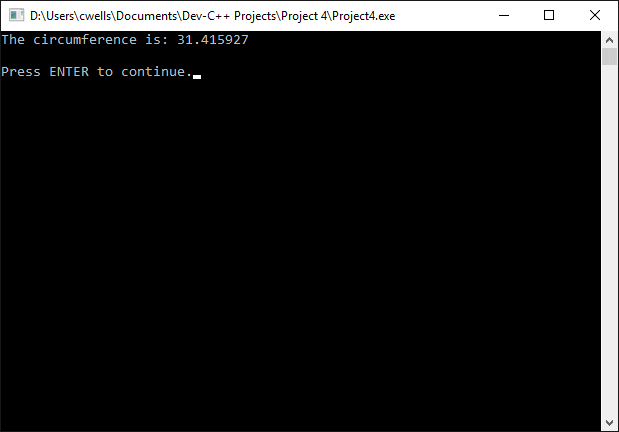# Constants

Constants are expressions that have a fixed value. Unlike variables, their value cannot be changed by the program code once they have been declared. Constants can be numeric, character values or strings. Numeric values can be integer or floating point values, and can also be expressed as octal (base 8) or hexadecimal (base 16) numbers. Constants can be defined using the const keyword as follows:

const double PI = 3.14159265;

In the example above, PI is the identifier that will be used in the program to represent the constant value 3.14159265. The following short program demonstrates the use of this constant.

#include <stdio.h>

const double PI = 3.14159265;

void main ()
{
double circ;     // circumference
char str;

circ = 2 * PI * rad;
printf( "The circumference is: %f", circ);
printf( "\n\nPress ENTER to continue.");
gets( str );
}

The compiler replaces any occurrence of the identifier PI in the program with the floating point value 3.14159265.The output from the example program